# Rosenbrock methods

A numerical integration method of the Runge–Kutta type for stiff systems of ordinary differential equations (cf. also Runge–Kutta method; Stiff differential system). For Rosenbrock methods these systems are usually put in the autonomous form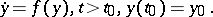(a1)

The property of stiffness, which means that the solutionis composed of components possessing widely differing time constants, impedes some form of implicitness for reasons of numerical stability [a1], [a2]. The most well-known implicit method for stiff initial-value problems is the backward Euler method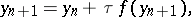(a2)

whereis the step size and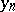the approximation to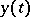at time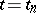. Since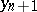is defined implicitly, this numerical solution itself must also be approximated. Mostly the iterative Newton method or a modification thereof is used, again for reasons of numerical stability. However, as the Euler method has only order of consistency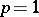, it makes no sense to carry out the iteration to high accuracy. In practice it often is sufficient to apply just one iteration per time step. If one then assumes thatis used as initial iterate, the following numerical result is found: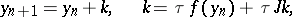(a3)

whereis the unit matrix and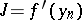denotes the Jacobian matrix of the vector functionat.

Of interest is that now the numerical solution is computed by solving a system of linear algebraic equations, rather than a system of non-linear equations. H.H. Rosenbrock, in his landmark paper [a3] of 1963, has proposed to generalize this linearly implicit approach to methods using more stages so as to achieve a higher order of consistency. The crucial consideration hereby is that no longer the iterative Newton method is used, but instead stable formulas are derived by working the Jacobian matrix directly into the integration formula. His idea has found widespread use. A generally accepted form in the literature for a so-called-stage Rosenbrock method is (cf. [a1], [a2]):(a4)Assuming that, hence equal for all stages, then one time step costs one Jacobian evaluation, one LU-decomposition of the matrixandbacksolves accompanied byfunction evaluations. Per step, Rosenbrock methods are therefore computationally expensive. Yet they are attractive since they are of one-step type, can be made A-stable or L-stable (cf. also Stability) and are easy to use since no iteration procedure as for genuinely implicit methods is required.

Rosenbrock methods are also called Runge–Kutta–Rosenbrock methods. If one puts, a classical explicit Runge–Kutta method results. Rosenbrock methods have been shown very valuable in practice. A good example is provided by the FORTRAN solver RODAS from [a2]. The underlying method of this solver uses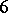stages, is L-stable and stiffly accurate and of order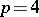. This solver is also applicable to linearly implicit systems of the form. In the literature, variants of the Rosenbrock method are discussed in which the Jacobian matrix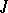is frozen for a number of time steps or even replaced by an approximation which renders the linear system solution cheaper (see [a1], [a2]). For very large-scale problems this is obviously of practical importance as it can reduce CPU time considerably.